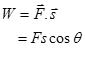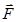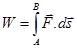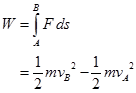Click to Chat

1800-1023-196

+91-120-4616500

CART 0

• 0

MY CART (5)

Use Coupon: CART20 and get 20% off on all online Study Material

ITEM
DETAILS
MRP
DISCOUNT
FINAL PRICE
Total Price: Rs.

There are no items in this cart.
Continue Shopping• Complete JEE Main/Advanced Course and Test Series
• OFFERED PRICE: Rs. 15,900
• View Details

Revision Notes on Work, Power and Energy

Work:-  Work done W is defined as the dot product of force F and displacement s.Here θ is the angle betweenand.

Work done by the force is positive if the angle between force and displacement is acute (0°<θ<90°) as cos θ is positive. This signifies, when the force and displacement are in same direction, work done is positive. This work is said to be done upon the body.

When the force acts in a direction at right angle to the direction of displacement (cos90° = 0), no work is done (zero work).Work done by the force is negative if the angle between force and displacement is obtuse (90°<θ<180°)  as cosθ is negative. This signifies, when the force and displacement are in opposite direction, work done is negative. This work is said to be done by the body.

Work done by a variable force:-

If applied force F is not a constant force, then work done by this force in moving the body from position A to B will be,Here ds is the small displacement.

Units:  The unit of work done in S.I is joule (J) and in C.G.S system is erg.

1J = 1 N.m , 1 erg = 1 dyn.cm

Relation between Joule and erg:- 1 J = 107 erg

Power:-The rate at which work is done is called power and is defined as,

P = W/t = F.s/v = F.v

Here s is the distance and v is the speed.

Instantaneous power in terms of mechanical energy:- P = dE/dt

Units: The unit of power in S.I system is J/s (watt) and in C.G.S system is erg/s.

Energy:-

1) Energy is the ability of the body to do some work. The unit of energy is same as that of work.

2) Kinetic Energy (K):- It is defined as,

K= ½ mv2

Here m is the mass of the body and v is the speed of the body.

Potential Energy (U):- Potential energy of a body is defined as, U = mgh

Here, m is the mass of the body, g is the free fall acceleration (acceleration due to gravity) and h is the height.

Gravitational Potential Energy:- An object’s gravitational potential energy U is its mass m times the acceleration due to gravity g times its height h above a zero level.

In symbol’s,

U = mgh

Relation between Kinetic Energy (K) and momentum  (p):-

K = p2/2m

If two bodies of different masses have same momentum, body with a greater mass shall have lesser kinetic energy.

If two bodies of different mass have same kinetic energy, body with a greater mass shall have greater momentum.

For two bodies having same mass, the body having greater momentum shall have greater kinetic energy.

Work energy Theorem:- It states that work done on the body or by the body is equal to the net change in its kinetic energy .

For constant force,

W = ½ mv2 – ½ mu2

= Final K.E – Initial K.E

For variable force,

?Law of conservation of energy:- It states that, “Energy can neither be created nor destroyed. It can be converted from one form to another. The sum of total energy, in this universe, is always same”.

The sum of the kinetic and potential energies of an object is called mechanical energy. So, E = K+U

In accordance to law of conservation of energy, the total mechanical energy of the system always remains constant.

So, mgh + ½ mv2 = constant

In an isolated system, the total energy Etotal of the system is constant.

So, E = U+K = constant

Or, Ui+Ki = Uf+Kf

Or, ?U = -?K

Speed of particle v in a central force field:

v = √2/m [E-U(x)]

Conservation of linear momentum:-

?In an isolated system (no external force ( Fext = 0)), the total momentum of the system before collision would be equal to total momentum of the system after collision.

So, pf = pi

Coefficient of restitution (e):- It is defined as the ratio between magnitude of impulse during period of restitution to that during period of deformation.

e = relative velocity after collision / relative velocity before collision

= v2 – v1/u1 – u2

Case (i) For perfectly elastic collision, e = 1. Thus, v2 – v1 = u1 – u2. This signifies the relative velocities of two bodies before and after collision are same.

Case (ii) For inelastic collision, e<1. Thus, v2 – v1 < u1 – u2. This signifies, the value of e shall depend upon the extent of loss of kinetic energy during collision.

Case (iii) For perfectly inelastic collision, e = 0. Thus, v2 – v1 =0, or v2 = v1. This signifies the two bodies shall move together with same velocity. Therefore, there shall be no separation between them.

Elastic collision:- In an elastic collision, both the momentum and kinetic energy conserved.

One dimensional elastic collision:-

?After collision, the velocity of two body will be,

v1 = (m1-m2/ m1+m2)u1 + (2m2/ m1+m2)u2

and

v2 = (m2-m1/ m1+m2)u2 + (2m1/ m1+m2)u1

Case:I

When both the colliding bodies are of the same mass, i.e., m1 = m2, then,

v1 = u2 and v2 = u1

Case:II

When the body B of mass m2 is initially at rest, i.e., u2 = 0, then,

v1 = (m1-m2/ m1+m2)u1 and v2 = (2m1/ m1+m2)u1

(a) When  m2<<m1, then, v1 = u1 and v2 = 2u1

(b) When  m2=m1, then, v1 =0  and v2 = u1

(c) When  m2>>m1, then, v1 = -u1 and v2 will be very small.

Inelastic collision:- In an inelastic collision, only the quantity momentum is conserved but not kinetic energy.

v = (m1u1+m2u2) /(m1+m2)

and

loss in kinetic energy, E = ½ m1u12+ ½ m2u22 - ½ (m1+ m2)v2

or,

E= ½ (m1u12 + m2u22) – ½ [(m1u1+ m2u2)/( m1+ m2)]2

= m1 m2 (u1-u2)2 / 2( m1 + m2)

Points to be Notice:-

(i) The maximum transfer energy occurs if m1= m2

(ii) If Ki is the initial kinetic energy and Kf is the final kinetic energy of mass m1, the fractional decrease in kinetic energy is given by,

Ki – Kf / Ki = 1- v12/u21

Further, if m2 = nm1 and u2 = 0, then,

Ki – Kf / Ki = 4n/(1+n)2

Conservation Equation:

(i)  Momentum – m1u1+m2u2 = m1v1+m2v2

(ii) Energy – ½ m1u12+ ½ m2u22 = ½ m1v12+ ½ m2v22

Conservative force (F):- Conservative force is equal to the negative gradient of potential V of the field of that force. This force is also called central force.

So,  F = - (dV/dr)

The line integral of a conservative force around a closed path is always zero.

So,

Spring potential energy (Es):- It is defined as,Es = ½ kx2

Here k is the spring constant and x is the elongation.

Equilibrium Conditions:

(a) Condition for equilibrium, dU/dx = 0

(b) For stable equilibrium,

U(x) = minimum,

dU/dx = 0,

d2U/dx2 = +ve

(c) For unstable equilibrium,

U(x) = maximum

dU/dx = 0

d2U/dx2 = -ve

(d) For neutral equilibrium,

U(x) = constant

dU/dx = 0

d2U/dx2 = 0

UNITS AND DIMENSIONS OF WORK, POWER AND ENERGY

Work and Energy are measured in the same units. Power, being the rate at which work is done, is measured in a different unit.

Quantity and  Units/Dimensions

Work (Energy)

Power

Dimension

ML2T-2

ML2T-3

Absolute

MKS

Joule

Watt

FPS

ft-Poundal

ft-poundal/sec

CGS

erg

Erg/sec.

Gravitational

MKS

kg-m

Kg-m/sec

FPS

ft-lb

ft-lb/sec.

CGS

gm-cm

Gm-cm/sec

Practical
(Other)

kwh, eV, cal

HP, kW, MW

Conversions between Different Systems of Units

1 Joule = 1 Newton ´ 1 m = 105 dyne ´ 102 cm = 107 erg

1 watt = 1 Joule/ sec = 107 erg/sec.

1 kwh  = 103 watt ´ 1 hr  = 103 watt ´ 3600 sec

= 3.6 ´ 106 Joule

1HP = 746 watt.

1 MW = 106 watt.

1 cal = 1 calorie = 4.2 Joule

1eV = "e" Joule  = 1.6 ´ 10-19 Joule

(e = magnitude of charge on the electron in coulombs)### Course Features

• 728 Video Lectures
• Revision Notes
• Previous Year Papers
• Mind Map
• Study Planner
• NCERT Solutions
• Discussion Forum
• Test paper with Video Solution